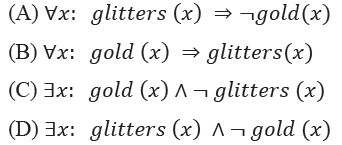# GATE | GATE-CS-2014-(Set-1) | Question 11

• Difficulty Level : Easy
• Last Updated : 28 Jun, 2021

Consider the statement

“Not all that glitters is gold”

Predicate glitters(x) is true if x glitters and predicate gold(x) is true if x is gold. Which one of the following logical formulae represents the above statement?(A) A
(B) B
(C) C
(D) D

Explanation:

The statement “Not all that glitters is gold” can be expressed as follows :

¬(∀x(glitters(x)⇒gold(x)) … (1)

Where ∀x(glitters(x)⇒gold(x) refers that all glitters is gold. Now ,

x¬(glitters(x)⇒gold(x)) … (2) , Since we know ¬∀x() = ∃x¬()

(Where ∀ refers to -> All and ∃x refers to -> There exists some).

As we know, A⇒B is true only in the case that either A is false or B is true. It can also defined in the other way :

A⇒B=¬A∨B (negationA or B ) … (3)

From equation (2) and (3) , we have

x(¬(¬glitters(x)∨gold(x))

⇒∃x(glitters(x)∧¬gold(x)) … (4) , Negation cancellation ¬(¬) = () : and ¬(()∨()) = (¬()∧¬()) .